## BREAK NEWS# Modern physic (A-level paper 1 section C) Revision questions and answers1. (a) (i) What are cathode rays? (01marks)

Cathode rays are streams of fast moving electrons

(ii) State two properties of cathode rays (01mark)

• They are negatively charged
• Travel in straight lines
• Travel with the same speed
• Affect photographic plates

(iii) Explain two disadvantages of using the discharge tube in producing cathode rays. (02marks)

• a discharge tube is operated at very high voltage which is not safe to handle
• gas required at very low pressure which may not be achieved practically

(b) With the aid of a diagram, describe Millikan’s experiment to determine the charge on an oil drop (07marks)• Separation between the plates P1 and P2 is measured
• Oil is sprayed through the hole in plate P1.
• Oil drops are ionized by friction and /or X-rays
• With no p.d applied a drop is selected and its terminal velocity v1 is calculated
• For known density ρ of oil, density σ of air and coefficient of viscosity, η of air, radius of the drop is calculated from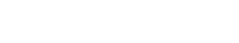• The p.d is now applied between the plates and varied until the selected drop remain stationary
• The p.d V is read and recorded.
• The charge Q carried by the drop is determined from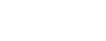(c) A beam of electrons is accelerated through a potential difference of 1.98kV and directed mid-way between two horizontal plates of length 4.8cm and separated by a distance of 2.0cm. The potential difference applied across the plates is 80.0V.

(i) Calculate the speed of the electrons as they enter the region between the plates (03marks)(ii) Explain the motion of the electrons between the plates (02marks)

• The horizontal velocity is unaffected because the net force is zero
• The vertical component of velocity changes with time due to electric force. Thus electron beam describes a parabolic path.

(iii) Find the speed of electrons as they emerge from the region between the plates (04marks)1. (a)(i) What is meant by thermionic emission? (01marks)

Thermionic emission is the ejection of electrons from metal surface when heated

(ii) Describe how full-wave rectification of a.c can be achieved using four semiconductor diodes. (04marks)• When X is positive relative to Y, diodes B and D conduct whereas diodes A and C do not.
• When Y is positive, diodes A and C conduct whereas B and D do not.
• In each case, the current through the load is in the same direction from N to M

(b) (i) Draw a labelled diagram to show the main parts of a cathode ray oscilloscope (C.R.O) (03marks)(ii) Describe how a C.R.O can be used as an a.c voltmeter. (02marks)

• Unknown a.c voltage V is connected to the Y-plates.
• The Y-sensitivity gain setting is adjusted to a suitable value V0 volts per centimetre
• When the time base is off, the vertical line on the screen is centered and its length L measured
• The unknown a.c voltage, V = V0L

(c) (i) an electron of charge –e and mass m moves in circular orbit round a central hydrogen nucleus of charge +e. Derive an expression for total energy of electron in an orbit of radius r. (05 marks)(ii) Why is this energy always negative (01marks)

The energy is always negative because the electron s bound to the nucleus by electrostatic force.

(d) (i) what is meant by excitation potential of an atom? (01marks)

Excitation potential of an atom is the potential required to raise atom from ground state to excited state.

(ii) Some of the energy levels in mercury spectrum are shown in the figure below.Calculate the wavelength of the radiation emitted when electron makes a transition from level A to level C. (03marks)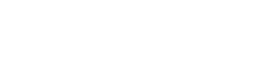1. (a) What is meant by the following as applied to radioactivity?

(i) Activity (01marks)

Activity is the number of atoms disintegrating per second

(ii) Half-life of a radioactive material (01marks)

Half-life is the time taken by the number of atoms to decay to half original value.

(b) Using the radioactive decay law N = N0eλt, show that the half-life  (02marks)(c) With the aid of a labelled diagram, describe the action of an ionization chamber. (05marks)• A radiation source on the brass flat form causes ionization of air in the chamber producing electrons and positive ions.
• The electrons move to the metal can and positive ions drift to the central metal rod.
• Movement of the ions to the electrodes causes discharge and current pulse flows in external circuit.
• The current sensitive detector detects current.
• The magnitude of current detected shows the extent to which ionization takes place.

(d) What is meant by unified atomic unit and electron volt? (02marks)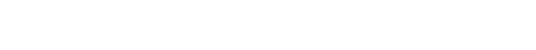Electron volt is the kinetic energy gained by electron which has been accelerated through a p.d of 1volt.(ii) Explain two uses of radioactive isotopes. (04marks)

• Carbon dating: activity of fresh and dad material samples are obtained and the age of the dead material obtained.
• Treatment of cancer, A dose of the radioactive isotope is administered to a patient. The isotope emits radiation which destroy cancer cells
• Detection of leakage in underground sewage and water pipes.
1. (a) Define the following

(i) Binding energy (01marks)

Binding energy is the energy required to split the nucleus into protons and neutrons.

(ii) Unified Atomic Energy (01marks)

Unified atomic mass unit is a twelfth of the mass of one atom of carbon-12 isotope.

(b) Explain how energy is released in a nuclear fusion process. (03marks)

When two small nuclei combine, the total mass of the two nuclei is greater than the mass of the resulting heavy nucleus. The difference in the mass accounts for the energy released.

(c) Explain what is observed in a discharge tube when the pressure is gradually reduced to low values? (05marks)(d) With the aid of a diagram, describe the operation of Bainbridge mass spectrometer in the determination of charge to mass ratio. (07marks)T1 and T2 are tracers on photographic plate, S1, S2 and S3 are slits

Mode of action

• Positive ions are produced in a discharge tube and admitted as a beam through slits S1 and S2.
• The beam then passes between insulated plates P, Q, connected to a battery, which create an electric field of intensity E.
• A uniform magnetic field B1, perpendicular to E is applied over the region of the plates and all ions, charge e with the same velocity, v given by B1ev =Ee will then pass undeflected through the plates and through a slit S3.
• The selected ions are deflected in a circular path of radius r by a uniform perpendicular magnetic field B2 and an image is produced on a photographic plate as shown.(e) An ion of mass 2.6 x 10-26 kg moving at a speed of 4 x 104ms-1 enter a region of uniform magnetic field of flux density 0.05T. Calculate the radius of the circle described by the ion.1. (a)(i) State three differences between X-rays and cathode rays. (03marks)

 X-rays Cathode rays Electrical magnetic wave of very short wavelength Streams of fast moving electrons Have no charge Are negatively charged Are not deflected by electric and magnetic field Are deflected by both magnetic and electric field Move with high speed Move with low speed Affect photographic plates Have no effect on photographic plates

(ii)Describe using a labelled diagram, the mode of operation of an X-ray tube (06marks)Mode of operation

• The filament is heated by a low voltage supply and the electrons are emitted by thermionic emission.
• The concave focusing cathode focuses the electrons from the filament onto the target.
• These electrons are accelerated towards the anode by the high voltage between the filament and the Anode.
• When the electrons (cathode rays) strike the metal target, about only 1% their kinetic energy is converted to X-rays and the 99% of their kinetic energy is converted to heat, which is conducted away by the cooling fins.

(iii)What is the difference between soft and hard X-rays (01mark)

• Hard X-rays have higher penetrating power than soft X-rays
• Hard X-rays have shorter wavelength than soft X-ray.

(b) (i) What is the main distinction between work function and ionization energy? (02marks)

Ionization energy is the minimum energy required to remove its most loosely bound electron from the atom while work function is the minimum energy required to liberate an electron from a metal surface.

(ii) An electron of charge, e, enters at right angles into a uniform magnetic field of flux density B and rotates at frequency, f, n a circle of radius, r.(c) An X-ray beam is produced when electrons are accelerated through 50kV are stopped by the target of an X-ray tube. When the beam falls on a set of parallel atomic plates of a certain metal at glancing angle of 160, a first order diffraction maximum occurs. Calculate the atomic spacing of the planes. (05marks)1. (a) State two differences between alpha and beta particles
 Alpha particle Beta particles Positively charged Negatively charged Low penetrating power High penetrating power High ionizing power Low ionizing power Helium nucleus An electron

(b) Describe with the aid of a diagram, the structure and mode of operation of an ionization chamber. (06marks)• A radiation source on the brass flat form causes ionization of air in the chamber producing electrons and positive ions.
• The electrons move to the metal can and positive ions drift to the central metal rod.
• Movement of the ions to the electrodes causes discharge and current pulse flows in external circuit.
• The current sensitive detector detects current.
• The magnitude of current detected shows the extent to which ionization takes place.

(c)(i) Explain the application of carbon-14 in carbon dating. (03marks)

• Carbon-14 isotope is radioactive with half-life t½ = 5600years
• It is absorbed and maintained at constant concentration by plants during photosynthesis.
• When plants die carbon- 14 decays and its concentration falls due to lack of renewal by photosynthesis.
• If N0 is the activity of fresh plant and N is the activity of dead plant after time t, the age of the dead plant t is deduced from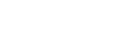(ii) A sample of dad wood was found to have activity of 20units due to carbon-14 isotope whose half-life is 5600 years. If activity of wood just cut is 47.8 units, estimate the age of the sample. (03marks)(d) The photoelectric work function of potassium is 2.25eV. Light having a wavelength of 360mm falls on a potassium metal.

(i) Calculate the stopping potential (04marks)(ii) Calculate the speed of the most energetic electron emitted by the metal (02marks)1. (a) What is meant by the following

(ii) Isotopes (01marks)

Isotopes are atoms of the same atomic number but difference atomic mass

(b) (i) Define mass defect  (01mark)

Mass defect is the difference in mass of constituent nucleons and the nucleus of an atom

(ii) State the conditions for a heavy nucleus to be unstable (01mark)

A heavy nucleus is unstable if there are too many neutrons or too many protons.

• Large number of protons increase electrostatic repulsion between themselves in a nucleus
• Large number of neutrons lead to unbalanced nuclear forces

(c) A sample of  emits both α-particles and γ-rays. A mass defect of 0.0053u occurs in the decay.

(i) Calculate the energy released in joules. (03 marks)

E =mc2 = 0.0053 x 1.66 x 10-27 x (3 x 108)2 = 7.92 x 19-13J

(ii) If the sample decays by emission of α-particle, each of energy 4.60MeV and γ-rays, find the frequency of the γ-rays emitted. (04marks)

4.60MeV = 4.60 x 106 x 1.6 x 10-19 = 7.36 x 10-13J

Energy of a photon of γ – rays = 7.9 x 19-13 – 7.36 x 10-13 = 0.56 x 10-13

From E = hf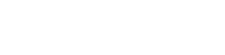(d) (i) Sketch a graph showing the variation of binding energy per nucleon with mass number, clearly showing the fusion and fissions. (02marks)(ii) Use the sketch in (d)(i) to explain how energy is released in each of the process of fusion and fission. (03marks)

• Two Small nuclei with atomic mass less than 56 each fuse to give a heavier nuclei with smaller mass by higher binding energy to increase stability of nucleon
• A nucleus with atomic mass higher than 56 split to form lighter nuclei of higher binding energy per nuclei.

(e) State two

• Treatment of cancer
• Production of energy in nuclear reaction
• Detection of links in pipes
• Carbon dating

(ii) health hazards of radioisotopes (01mark)

• Cause genetic mutation
• Cause cancer
• Cause deep seated wounds in humans
1. (a) What are X-rays? (01marks)

X- rays are electromagnetic radiations of very high frequency (short wavelength) produced when cathode rays strike metal target.

(b) (i) With the aid of a diagram explain how X-rays are produced in an X-ray tube (05marks)Mode of operation

• The filament is heated by a low voltage supply and the electrons are emitted by thermionic emission.
• The concave focusing cathode focuses the electrons from the filament onto the target.
• These electrons are accelerated towards the anode by the high voltage between the filament and the Anode.
• When the electrons (cathode rays) strike the metal target, about only 1% their kinetic energy is converted to X-rays and the 99% of their kinetic energy is converted to heat, which is conducted away by the cooling fins.

(ii) State the energy changes that take place in the production of X-rays in an X-ray tube. (02marks)

Electrical energy → kinetic energy → X-rays + heat

(c) In an X-ray tube, the electrons strike the target with a velocity of 3.75 x 107ms-1 after travelling a distance of 5.0cm from the cathode. If a current of 10mA flows through the tube, find the

(i) tube voltage (02marks)(ii) number of electrons striking the target per second. (02marks)(iii) Number of electrons within the space of 1cm length between the anode and the cathode. (05marks)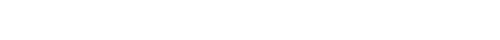(d) Briefly explain one medical application of x-rays (03marks)

X-rays are used to kill cancer cells

1. (a) (i) Distinguish between mass defect and binding energy of an atomic nucleus (01mark)

Mass defect s the difference between the mass of nucleons that make up a nucleus and the mass of a nucleus.

Binding energy of an atomic nucleus is the minimum energy to break up a nucleus into its constituent nucleons.

(ii) Sketch a graph of nuclear binding energy per nucleon versus mass number for naturally occurring isotopes and use it to distinguish between nuclear fission and fusion. (04marks)• Two Small nuclei with atomic mass less than 56 each fuse to give a heavier nuclei with smaller mass by higher binding energy to increase stability of nucleon
• A nucleus with atomic mass higher than 56 split to form lighter nuclei of higher binding energy per nuclei.

(b) Describe with the aid of a diagram, Millikan’s oil drop experiment to determine charge on oil drop. (07marks)• Separation between the plates P1 and P2 is measured
• Oil is sprayed through the hole in plate P1.
• Oil drops are ionized by friction and /or X-rays
• With no p.d applied a drop is selected and its terminal velocity v1 is calculated
• For known density ρ of oil, density σ of air and coefficient of viscosity, η of air, radius of the drop is calculated from• The p.d is now applied between the plates and varied until the selected drop remain stationary
• The p.d V is read and recorded.
• The charge Q carried by the drop is determined from(c) (i) Explain briefly diffraction of X-rays by crystals and derive Bragg’s law. (06marks)

A parallel beam of monochromatic X-rays incident on a crystal is reflected from successive atomic planes and super-imposed, forming an interference patternsFor constructive interference to occur, the path difference is equal to the whole number of wavelength

Thus BC + CD = nλ

dsinθ + dsinθ = nλ

or 2dsinθ = nλ  where n = 1, 2, 3, 4 …

(ii) A second order diffraction image by reflection of X-rays at atomic plates of crystal for glancing angle of 11024’. Calculate the atomic spacing of the plates if the wavelength of X-ray is 4.0 x 10-11m. (02marks)1. (a) state Bohr’s postulates of an atom (03marks)

Bohr’s model state that electrons move in allowed orbits round the nucleus and while in orbits, they do not radiate any energy

(b) An electron of mass m and charge –e, is considered to move in circular orbit about a proton.

(i) Write down the expression for the electric force on the electron. (02marks)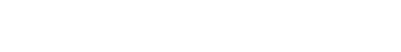(ii) Derive an expression for total energy given that the angular momentum for the electron is(c) With the aid of a labelled diagram, describe the operation of a diffusion type cloud chamber. (06marks)• The base of the chamber is maintained at low temperature, about -800c by the solid carbon dioxide while the top of the chamber is at room temperature, and so there is a temperature gradient between the top and the bottom of the chamber.
• The air at the top of the chamber is saturated with alcohol vapor from the felt ring. This vapor continuously diffuse downwards into the cooler regions so that the air at the chamber is super saturated with alcohol vapor.
• Radiations from the radioactive source S cause the ionization of the vapor.
• The ionizations from the radioactive source S cause condensation of the vapor on the ions formed, hence the path of the ionizing radiations are traced by series of small droplets of condensation.
• The thickness and length of the path indicate the extent to which ionization has taken place.
• Alpha particles produce short, thick, continuous straight tracks
• Beta particles which are less massive produce longer, thin but straggly paths owing to collisions with gas molecules
• Gamma radiations are uncharged and for ionization to take place, it must collide with an atom and eject an electron which then ionizes the vapor.

(d) The energy levels of an atom have values

E1 = – 21.4eV

E2= -4.87 eV

E3 = -2.77 eV

E4 = -0.81eV

E = 0.00eV

(d) Calculate the wavelength of radiation emitted when an electron makes a transition from E3 to E2 (03marks)(ii) State the region of the electromagnetic spectrum where the radiation lies (01mark)

Visible spectrum

11. (a) Describe how positive rays are produced. (03marks)Positive rays are produced when a stream of electrons is passed through a vapor (gas) in discharge tube. The electrons dislodge electrons from the atoms producing positively charged ions.  The positive ions are accelerated towards perforated cathode.  The ions pass through the slits and are further accelerated. These ions constitute a stream of positive rays.

(b) Describe how a Bainbridge spectrometer can be used to detect isotopes. (05marks)• Positive ions are directed through slits S1 and S2.
• The ions enter a velocity selector carrying crossed electric and magnetic field.
• Selected ions enter a region of uniform magnetic field in circular paths and strike the photographic plate at different points

(c) (i) What is time base as applied to a Cathode Ray Oscilloscope? (01mark)

Is a circuit connected to the x-plate of cathode ray oscilloscope to sweep the electron beam across a screen of a C.R.O

(ii) Draw a sketch graph showing the variation of time base voltage with time (01mark)(d) An alternating p.d applied to the Y-plate of an oscilloscope produces five complete waves on a 10cm length of the screen when the time base setting is 10mscm-1. Find the frequency of the alternating voltage. (03marks)(e) (i) Explain the motion of an electron projected perpendicularly into a uniform magnetic field. (03marks)

By Fleming’s left hand rule, the electrons experience a force which is always at right angles to the magnetic field and the direction of motion. The speed of electrons remain unaltered but the electrons is deflected by from its original path by this force. The motion of electrons in a magnetic field is a circular path.

(ii) An electron accelerated from rest by a p.d of 100V, enters perpendicularly into a uniform electric field intensity 105Vm-1. Find the magnetic field density, B, which must be applied perpendicularly to the electric field so that the electron passes undeflected through the fields. (04marks)Avogadro’s constant is the number of atoms in one mole of substance.

Faradays constant is the charge required to liberate one mole of monovalent ions during electrolysis.

(ii) Show that the charge carried by a monovalent ion is 1.6 x 10-19C. (02marks)

1F = nq (q = electronic charge)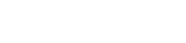(b) With the use of a labelled diagram, describe Millikan’s oil drop experiment for the determination of the charge of an electron. (07marks)

Millikan’s Oil drop experiment

This is used to determine electronic charge eProcedure

Procedure

– Set up of the apparatus is as shown above

– Oil drops are introduced between the plates P1 and P2 by spraying using the atomizer.

– These oil drops are charged in the process of spraying by friction but the charge may be increased further ionization due to X-rays.

– The oil drops are strongly illuminated by an intense light from the arc lamp so that they appear as bright spots when observed through a low power microscope.

– With no electric field between the plates, record the time t1 taken for drop to fall from P1 to P2.

– The electric field between the plates is turned on and adjusted so that the drop becomes stationary.

Case 1

With no electric field, the oil drop falls with a uniform velocity v1 called terminal velocityCase 2

When the electric field is applied so that the drop is stationary, the drop has no velocity and no acceleration.(c) A beam of positive ions moving with velocity v enters a region of a uniform magnetic field density B with the velocity at right angles to the field B. By use of a diagram, describe the motion of ions. (03marks)(d) A charged oil drop of density 880kgm-3 is held stationary between two parallel plates 6.0mm apart held at a potential difference of 103V. When the electric field is switched off, the drop is observed to fall a distance of 2.0mm in 35.7s. (Viscosity of air = 1.8 x 10-5Nsm-2, Density of air = 1.29kgm-3).

(i) Calculate the radius of the drop. (03marks)(ii) Estimate the number of excess electrons on the drop. (03marks)13. (a) (i) State the laws of photoelectric emission (04marks)

• The time lag between irradiation of the metal surface and emission of the electrons by the metal surface is negligible.
• For a given metal, surface there is a minimum value of frequency of radiation called threshold frequency (f0) below which no photo electrons are emitted from the metal however intense the incident radiation may be.
• The number of photoelectrons emitted from the surface per second is directly proportional to the intensity of incident radiation for a particular incident frequency
• The K.E of the photoelectrons emitted is independent of the intensity of the incident radiation but depends only on its frequency

(ii) Explain briefly one application of photoelectric effect. (04marks)

Use in a photocell in a burglar alarm: when an intruder intercepts infrared radiation incident on the photocell, the flow of current is interrupted. The alarm is set off

(b) In a photoelectric set up. A point source of light of power 3.2 x 10-3W emits mono-energetic photons of energy 5.0eV. The source is located at a distance of 0.8m from the center of a stationary    metallic sphere of work function 3.0eV and radius 8.0 x 10-3m. The efficiency of photoelectron emission is one in every 106 incident photons.

Calculate

(i) Number of photoelectrons emitted per second. (04marks)(ii) Maximum kinetic energy in joules, the photo electrons. (02marks)

Maximum kinetic energy = 5- 3 = 2eV = 2 x 1.6 x 10-19 = 3.2 x 10-19J

(c) (i) State Bragg’s law of X-ray diffraction (01marks)

It states that for constructive interference of diffracted X-rays to occur, the path difference s an integral multiple of the wavelength of the X-ray.

Or

2dsinθ = nλ

Where  d = interatomic spacing

θ = glancing angle

λ = wavelength of X-rays

n = integral number

(ii) Show that density, ρ, of a crystal can be given bywhere θ is the glancing angle, n, is the order of diffraction, λ is the X-ray wavelength and M is the molecular weight of the crystal. (05marks)14. (a) With reference to a Geiger-Muller tube, define the following

(i) quenching agent (01mark)

It is a halogen gas placed in a Geiger-Muller tube to prevent positive ions from causing the release of                               electrons from the cathode.

(ii) back ground count rate (01mark)

It is the activity detected by the GM tube in absence of the radioactive source.

(b) (i) With the aid of a labelled diagram, describe the operation of a Geiger-Muller (GM) tube (06marks)The thin mica window allows the passage and detection of the weak penetrating alpha particles. The GM tube is first evacuated then filled with Neon, Argon plus Halogen gas which is used as a quenching agent.

Mode of operation

• When an ionizing particle enters the tube through the window, argon atoms are ionized.
• The electrons move to the anode while the positive ions drift to the cathode.
• A discharge occurs and the current flows in the external circuit.
• A p.d is obtained across a large resistance, R which is amplified and passed to the scale.
• The magnitude of the pulse registered gives the extent to which ionization occurred.

(ii) Explain how the half-life of a short lived radioactive source can be obtained by use of a Geiger-Muller tube. (04marks)

• Switch on the GM-tube, note and record the background count rate, A.
• Place a source of ionizing radiation near the GM-window.
• Note and record the count rate recorded the count rate at equal intervals.
• For each count rate recorded subtract the background count rate to get the true rate.
• Plot a graph of the count rate against time.Find time t1 taken for the activity to reduce to A/2 and t2 taken for activity to reduce to A/4 from A/2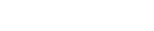(c) A radioactive isotope  which has a half-life of 14.3days, disintegrates to form a stable product. A sample of the isotope is prepared with initial activity of 2.0 x 106s-1. Calculate the

(Assume N = N0e-λt)

(i) the number of 32P atoms present (03marks)(ii) activity after 30days (03marks)(iii)  number of 32P atoms after 30 days (02marks)15. (a) State Rutherford’s model of the atom. (02marks)

The positive charge of an atom and nearly all the mass is concentrated in a small volume at the center. Electrons are in motion in circular orbits around the nucleus and the volume of the atom is accounted for by the electron cloud.

(b) Explain how Bohr’s model of the atom addresses the two main failures of Rutherford’s model. (07marks)

• Orbiting electrons experience centripetal acceleration. Therefore they continuously emit electromagnetic radiation hence would lose energy. This therefore implies that the electrons would spiral towards the nucleus and the atom ceases to exist. Yet the atom is stable Thus Rutherford model cannot explain the stability of the atom
• Since electron continuously accelerating around the nucleus, a continuous emission spectra should be emitted by the atom. However experimental observation reveals that it is atomic emission spectrum
• From Bohr’s model, electrons move around the nucleus in circular orbits. In these orbits the electron does not radiate energy. Electromagnetic radiation is emitted when the electron makes a transition between orbits
• The electron can only move in allowed orbits in which their angular momentum is equal to nh/2π

(c) With the aid of a labelled diagram, describe how cathode rays are produced. (05marks)• Hot Cathode C produces electrons by thermionic emission.
• The electrons are accelerated by p.d between cathode C and anode A

(d) (i) What is binding energy of a nucleus? (01mark)

Binding energy is the energy released when a nucleus is formed from its components (protons and neutrons)

(ii) Calculate the energy in MeV released by fusing four protons to form an alpha particle and two beta particles.

Mass of beta particle = 0.000549u

Mass of hydrogen atom = 1.007825u

Mass of helium atom = 4.002664u

[1u = 931MeV]                     (05marks)

Solution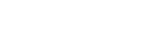Mass of the reactant = 4 x 1.00782 = 4.031300

Mass of the products = 4.002664 + 2 x 0.000549= 4.003762

Decrease in mass= 4.0031300 – 4.003762 =0.027538U

= 0.027538 x 931

= 25.64MeV

16. (a) What is photo electric emission? (01mark)

Is the emission of electrons from a clean surface of a metal when irradiated by electromagnetic radiation of light

(b)(i) Describe an experiment to demonstrate photo electric effect. (04marks)• Freshly cleaned zinc plate is placed on top of a negatively charged electroscope.
• Ultraviolet radiation is directed onto the zinc plate
• The leaf gradually falls indicating the electroscope has lost charge which are electrons.

(ii) When a clean surface of a metal in a vacuum is irradiated with light of wave length 5.5 x 10-7m, electrons just emerge from the surface. However when light of wavelength 5 x 10-7m is incident on the metal surface, electrons are emitted each with energy 3.62 x 10-20J. Find Plank’s constant. (04marks)(c) (i) With the aid of a labelled diagram, describe an X-ray tube and how X-rays are produced. (05marks)Mode of operation

• The filament is heated by a low voltage supply and the electrons are emitted by thermionic emission.
• The concave focusing cathode focuses the electrons from the filament onto the target.
• These electrons are accelerated towards the anode by the high voltage between the filament and the Anode.
• When the electrons (cathode rays) strike the metal target, about only 1% their kinetic energy is converted to X-rays and the 99% of their kinetic energy is converted to heat, which is conducted away by the cooling fins.

(ii) Describe how the intensity and quality of X-rays is controlled in an X-ray tube. (02marks)

• Intensity of X-rays is controlled by varying the filament current
• Quality of X-rays is controlled by the high voltage

(d) An X-ray tune operates at 1.5 x 10-3V and the current through it is 1.0 x 10-3A.

Find the

(i) number of electrons crossing the tube per second. (02marks)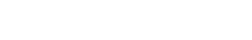(ii) kinetic energy gained by electron traversing the tube (02marks)

K.E = eV 1.6 x 10-19 x 1.5 x 10-3 = 2.4 x 10-16J

17. (a)(i) What is specific charge? (01mark)

Specific charge is the ratio of charge to mass of a particle

(ii) State the unit of specific charge (01mark)

Unit = Ckg-1

(iii) Describe with the aid of a diagram how the specific charge of positive ions can be determined using a mass spectrometer. (06marks)T1 and T2 are tracers on photographic plate, S1, S2 and S3 are slits

Mode of action

• Positive ions are produced in a discharge tube and admitted as a beam through slits S1 and S2.
• The beam then passes between insulated plates P, Q, connected to a battery, which create an electric field of intensity E.
• A uniform magnetic field B1, perpendicular to E is applied over the region of the plates and all ions, charge e with the same velocity, v given by B1ev =Ee will then pass undeflected through the plates and through a slit S3.
• The selected ions are deflected in a circular path of radius r by a uniform perpendicular magnetic field B2 and an image is produced on a photographic plate as shown.(b) A beam of strongly ionized carbon atoms passes undeflected through a region of crossed magnetic and electric field of 0.10T and 1.0 x 104NC-1 respectively. When it enters a region of uniform magnetic field, it is deflected through an arc of radius 0.75m. Calculate the magnetic flux density of this magnetic field. (Mass of carbon atom = 2.0 x 10-26kg)                (05marks)(c) (i) Draw a graph to illustrate the variation of ionization current and p.d across an ionization chamber and explain its features. (03marks)Region OA:

Current detected increases gradually but p.d is not large enough to prevent recombination of the ions.

Region AB. (saturation region)

Current is almost constant, all ions reach the electrode before recombination but there is no secondary ionization.

Region BC (gas amplification)

Current increases rapidly for small increase (change) in p.d. because secondary ionization takes place due to primary ions being produced. This implies many ion pairs, thus a larger current detected.

(ii) Explain how ionization chamber can be used to detect ionization radiation (04marks)• A radiation source on the brass flat form causes ionization of air in the chamber producing electrons and positive ions.
• The electrons move to the metal can and positive ions drift to the central metal rod.
• Movement of the ions to the electrodes causes discharge and current pulse flows in external circuit.
• The current sensitive detector detects current.
• The magnitude of current detected shows the extent to which ionization takes place.

1. (a) Explain briefly how positive rays are produced (03marks)• Positive rays are produced when a stream of electrons is passed through a vapor (gas) in discharge tube.
• The electrons dislodge electrons from the atoms producing positively charged ions.
• The positive ions are accelerated towards perforated cathode.
• The ions pass through the slits and are further accelerated.
• These ions constitute a stream of positive rays.

(b) An electron of charge, e, and mass, m, is emitted from a hot cathode and then accelerated by an electric field towards the anode. If the potential difference between the cathode and the anode is V, show that the speed of the electron, u, is given by(c) An electron starts from rest and moves in an electric field intensity of 2.4 x 103Vm-1.

Find the

(i) force on the electron (02 marks)

Force, F, on electron

F = eE = 1.6 x 10-19 x 2.4 x 103 = 3.84 x 10-16N

(ii) acceleration of the electron. (02marks)

From F =ma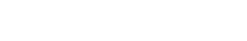(iii) velocity acquired in moving through a p.d of 90V (02marks)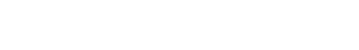(d)  A beam of electrons each of mass, m, and charge, e, is directed horizontally with speed, u, into an electric field between two horizontal metal plates separated by a distance, d.

(i) If the p.d between the plates is V, show that the deflection y of the beam is given by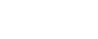where, x, is the horizontal distance travelled. (06marks)

Horizontal motion, x =ut

In the same time, t, vertical displacement(ii) Explain the path of the electron beam as it emerges out of the electric field. (02marks)

The electron beam continues in a straight path with constant velocity since the electric force on it is zero.

19. (a) The table below shows the energy levels of a hydrogen atom.

 Principal quantum number, n Energy, eV 6 -0.38 5 -0.54 4 -0.85 3 -1.51 2 -3.39 1 -13.60

(i) Why are the energies for the different levels negative? (01mark)

Work is done in order to remove an electron to infinity.

(ii) Calculate the wavelength of the line arising from a transition from the third to the second level. (03marks)(iii) Calculate the ionization energy in joules of hydrogen atom. (02marks)

Ionization energy = 13.6 x 1.6 x 10-19 = 2.18 x 10-18J

(b) Explain the physical processes in an X-ray tube that account for

(i) cut off wavelength (03marks)

Electrons from the cathode strike the target and lose all their kinetic energy in single encounter with the target atoms. This results in the production of the most energetic X-rays photon of maximum frequency and corresponding minimum wavelength (λmin) called the cut off wavelength.

(ii) characteristic lines (04marks)

At high tube voltages, the bombarding electron penetrate deep into the atom and knock out the electron from inner shell. The knocked out electron can either be ejected completely out of the atom or it occupies any of the higher shell. This puts an atom in an excited state and the atom therefore becomes unstable. Electron transition from higher shell to a vacancy left in the lower shell results in emission of an X-ray photon of energy equal to the difference between the energy levels.

(c) Calculate the maximum frequency of radiation emitted by an X-ray tube using an accelerating voltage of 33.0kV (03marks)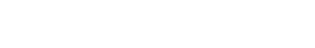(d) Derive Bragg’s law of X-ray diffraction in crystals. (04marks)

A parallel beam of monochromatic X-rays incident on a crystal is reflected from successive atomic planes and super-imposed, forming an interference patternsFor constructive interference to occur, the path difference is equal to the whole number of wavelength

Thus BC + CD = nλ

dsinθ + dsinθ = nλ

or 2dsinθ = nλ  where n = 1, 2, 3, 4 …

20. (a) A beam of α-particles is directed normally to a thin metal foil

Explain why

(i) Most of the α-particles passed straight through the foil (02marks)

The atoms of the foil contain concentrated mass in a very tiny nuclei surrounded by empty space containing electrons

(ii) Few α-particles are deflected through angles more than 900. (02marks)

The nucleus a very small space of an atom. Therefore very few α-particles are incident close to it that  are strongly repelled.

(b) Calculate the least distance of approach of a 3.5MeV α-particles to the nucleus of a gold atom. (Atomic number of gold= 79) (04marks)

Initial K.E = eV= P.E at the closest distance of approach; Z = 79(c) (i) Define space charge as applied to thermionic diodes. (01mark)

Space charge is cloud of negative charge around the cathode at low anode potential difference.

(ii) Draw anode current-diode voltage curves of a thermionic diode for two different filament currents and explain their main features. (06marks)The current increases with the positive anode potential as far as the point S. Beyond this point the current does not increase, because the anode is collecting all the electrons emitted by the filament ; the current is said to be saturated.

(d) (i)What is a decay constant?

Decay constant is the fractional number of disintegrations per second.

(ii) A sample from fresh wood of a certain species of tree has activity of 16.0 counts per minute per gram. However, the activity of 5g of dead wood of the same species of tree is 10 counts per minute. Calculate the age of the deadwood. (Assume half-life of 5730years) (04marks)

From N = N0e-λt